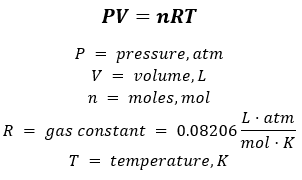# Problem: At standard temperature and pressure the molar volumes of Cl2 and NH3 gases are 22.06 and 22.40 L, respectively.Given the different molecular weights, dipole moments, and molecular shapes, why are their molar volumes nearly the same?

###### FREE Expert Solution

Assuming that Cl2 and NH3 are ideal gases, we will answer this problem using the ideal gas equation:Rearranging the equation for molar volume:

88% (227 ratings)###### Problem Details

At standard temperature and pressure the molar volumes of Cl2 and NH3 gases are 22.06 and 22.40 L, respectively.

Given the different molecular weights, dipole moments, and molecular shapes, why are their molar volumes nearly the same?